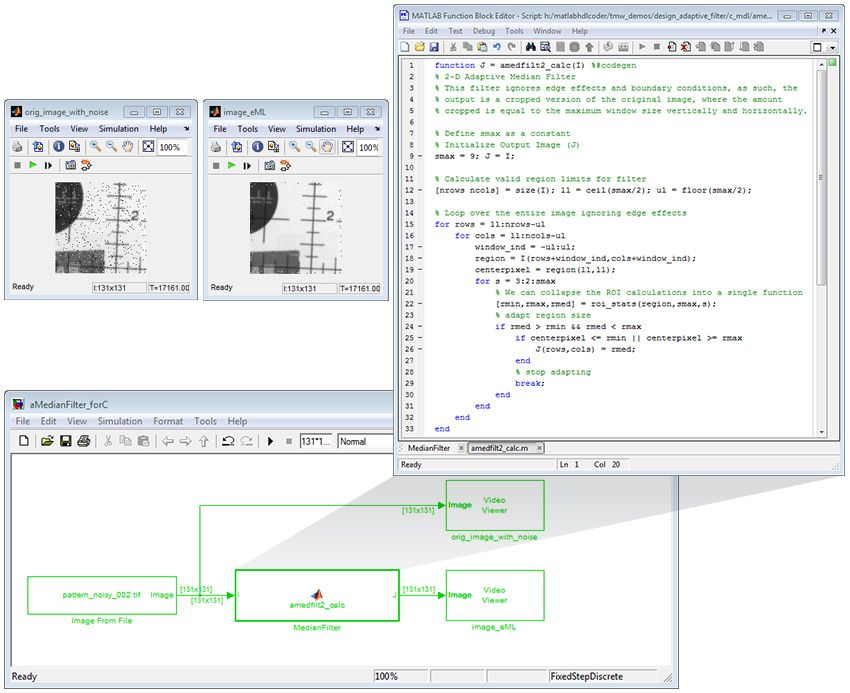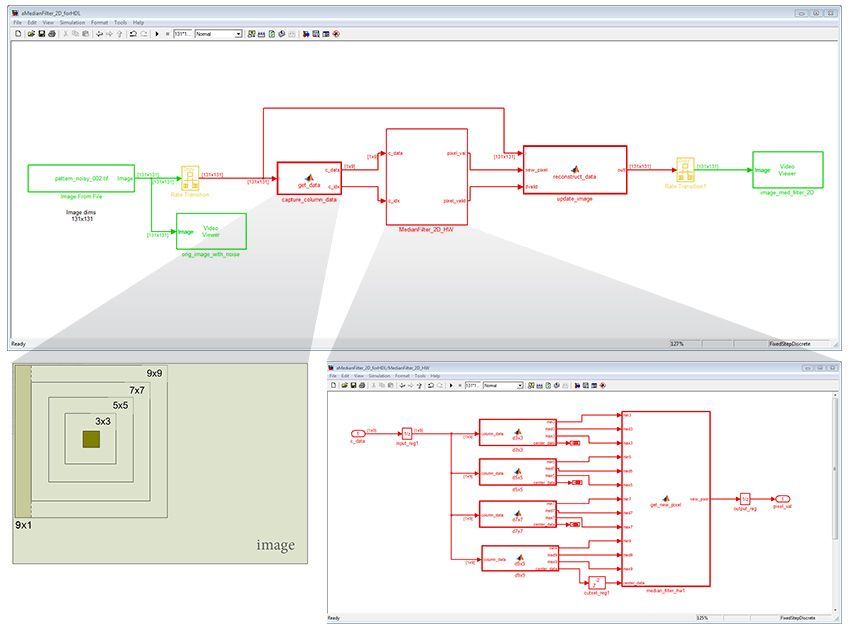# Converting MATLAB Algorithms into Serialized Designs for HDL Code Generation

By Kiran Kintali, MathWorks

Simulink® lets you integrate MATLAB® algorithms into a Simulink model for C or HDL code generation. However, many MATLAB implementations of signal processing, communications, and image processing algorithms require some redesign to make them suitable for HDL code generation. For example, they often use data types such as doubles, strings, and structures, and contain control flow constructs, such as while loops and break statements, that do not map well to hardware. Apart from these constructs, MATLAB algorithms that operate on large data sets are not always written to take account of hardware design characteristics like streaming and resource sharing. This article uses a typical software implementation of an adaptive median filter to illustrate the process of converting MATLAB algorithms for HDL code generation.

We start with a Simulink model that takes a noisy 131x131 pixel image and applies an adaptive median filter to obtain the denoised image (Figure 1, top left).

The current version of the algorithm is implemented in MATLAB for C code generation (Figure 1, top right). The algorithm takes the whole input image, 'I', as input, operates on the data in double precision, and returns the denoised image, 'J', as output. The core of the algorithm is implemented in three levels of nested loops operating on the entire image. The two outer loops iterate over the rows and columns of the image. The innermost loop implements the adaptive nature of the filter by comparing the median to a threshold and deciding whether to replace the pixel or increase the neighborhood size and recalculate the median.Figure 1. Simulink model of an adaptive median filter, set up for C code generation, with original and output images (top left) and corresponding MATLAB code (top right).

The algorithm uses four neighborhood sizes: 3x3, 5x5, 7x7, and 9x9. Even though the current implementation contains constructs and paradigms typical in software implementations and is efficient for software, in its current form it is not suitable for hardware synthesis, for the following reasons:

The algorithm operates on the entire image. Typical hardware implementations stream the data into the chip in small chunks called windows or kernels to reduce the chip I/O count; the data is processed at a faster rate, and the chip finishes processing an entire frame of data before the next frame is available.

The algorithm uses double data types. Double data types are not efficient for hardware realization. Hardware implementations must use silicon area efficiently and avoid usage of double-precision arithmetic, which consumes more area and power. The algorithm should use fixed-point data types as opposed to floating-point data types (double).

The algorithm uses expensive math functions. The use of operators like sin, divide, and modulo on variables leads to inefficient hardware. Naïve implementation of these functions in hardware results in low clock frequencies. For hardware design tradeoffs, we need to use low-cost repetitive add- or subtract-based algorithms, such as CORDIC.

Software loops in the algorithm must be mapped efficiently to hardware. Since hardware execution needs to be deterministic, we cannot allow loops with dynamic bounds. Hardware is parallel, which means that we could unroll the loop execution in hardware to increase concurrency, but this uses up more silicon area.

The algorithm contains large arrays and matrices. When mapped to hardware, large arrays and matrices consume area resources like registers and RAMs.

In the next section we will see how a restructured hardware-oriented implementation of the same adaptive median filter addresses each of these issues.

## Modifying the Adaptive Filter Algorithm for Hardware

The process of converting the original adaptive median algorithm to hardware involves the following tasks:

• Serializing the input image for processing
• Separating the adaptive median filter computation for parallelization
• Updating the original image using the denoised pixel values

## Serializing the Input Image

Most hardware algorithms do not work on the whole image but on smaller windows at each time step. As a result, the original input image must be serialized and streamed into the chip and, depending on how much of the image needs to be available for the algorithm computation, buffered onto the on-chip memory. This hardware modeling of the algorithm must take into account the amount of memory available to hold the image data, in addition to the number of I/O pins available on the chip to stream the data in.

In our example, serialization involves restructuring the Simulink model so that our adaptive filter design breaks the image into 9x1 columns of pixel data and feeds it as input to the filter. The data is buffered inside the chip for 9 cycles, creating a 9x9 window to compute a new center pixel. The filter processes this window of data and streams a modified center pixel value for the 9x9 window. At the output of the filter, the modified center pixel data is applied to the original image to reconstruct a denoised image. Now that the filter is working on smaller windows of data, it needs to run at a faster rate to finish processing the whole algorithm on the image before the next image is available at the input. We model this algorithm behavior using rate transition blocks.

This sort of image buffering would require additional control signals for the streaming of data to be processed by the algorithm implemented in the hardware. In this model (Figure 2) the subsystem "capture_column_ data" helps to sweep through the image, and in 9x1 windows, feeds the data to the main Filter subsystem ("MedianFilter_2D_ HW"). Since the 2D adaptive median filter works on a maximum window size of 9x9, it takes 9 cycles to fill the filter pipeline at the beginning of each row of images and compute the first center pixel. This means we need additional control signals at the output of the filter to indicate the validity of the center pixel output.Figure 2. Simulink model of an adaptive median filter, set up for HDL code generation, with representation of sweeps of 9x1 column of data by the "capture_column_data" subsystem (bottom left) and implementation of the adaptive median filter (bottom right).

At the output of the filter, the subsystem "update_image" takes the filtered data from the "MedianFilter_2D_HW" subsystem and reconstructs the full image based on the control signals.

## Parallelizing the Algorithm

The adaptive median filter bases its selection of a window size for calculating the median on local statistics. The software-oriented implementation computes these statistics for each window size sequentially in nested loops. The hardware implementation can perform these computations in parallel.

The new filter implementation partitions the data buffer into 3x3, 5x5, 7x7, and 9x9 regions and implements separate median filters to compute the minimum, median, and maximum values for each subregion in parallel (Figure 2, bottom right). Parallelizing the window computations lets the filter perform faster in hardware.

## Optimizing the Algorithm for Hardware

To find the minimum, median, and maximum values of the neighboring pixels, the nested loops in the software implementation iterate over all the rows from left to right and top to bottom. In the hardwarefriendly implementation, min/max/median computation occurs only on the regions of interest, identified using a 1D median filter. Figure 3 (top) shows computation of min/max/median values for a 3x3 window; as can be seen, an nxn region of pixels requires `{N2 * floor(log2 N2/2)}` number of comparators.

To implement the algorithm on 3x3, 5x5, 7x7, and 9x9 windows, we would require a total of 4752 (9*4 + 25*12 + 49*24 + 81*40) comparators.

We can explore other area tradeoffs—for example, we can implement a 2D filtering algorithm that works on individual rows and columns of the nxn region rather than on all the pixels. This would consume fewer resources than the 1D filter and would require 800 comparators (18 + 100 + 196 + 486) instead of 4752. However, because we know that the center pixel values are usually found in the 3x3 region, we could compromise on quality by applying the lossy 2D algorithm (`get_median_2d`) on other regions while applying the 1D algorithm on the 3x3 region (Figure 3, bottom).

To experiment with these tradeoffs we simply swap the call to functions on the path `get_median_1d` with `get_ median_2d` and simulate the model to compare the noise reduction differences between different choices.

The output pixel of this algorithm is used to denoise the original image.

MATLAB and Simulink provide a concise way of representing the algorithm: The adaptive median filter is described in about 186 lines of MATLAB code. A comparable C-code implementation would require almost 1000 lines; an HDL implementation, more than 2000 lines. Understanding the modeling tradeoffs when targeting hardware and software is key for efficient implementation of complex signal and video processing algorithms. MATLAB and Simulink help you to explore these tradeoffs at a high level of abstraction without encoding too much hardware detail, providing an effective way to use the MATLAB environment for hardware deployment.

Adaptive median filtering is a digital image processing technique commonly used to reduce speckle noise and saltand- pepper noise. A generic median filter replaces the current pixel value with the median of its neighboring pixel values; it affects all the pixels, whether or not they are noisy, and hence, blurs images with high noise content. The adaptive median filter overcomes this limitation by selectively replacing the pixel values. It makes the decision by analyzing the median. If the median is skewed by the noise, it adapts itself by defining the median over larger regions.

Because of these advantages, adaptive median filters are commonly used as a preprocessing step for cleaning up the image for further processing. Hardware implementations of the filters are highly desirable due to the algorithm's computation complexity and high throughput requirements.

Published 2011 - 91893v00